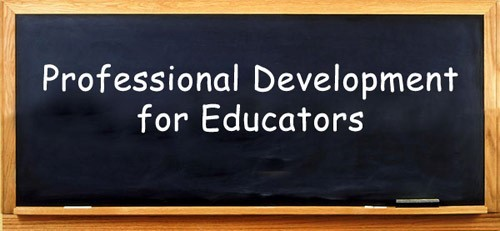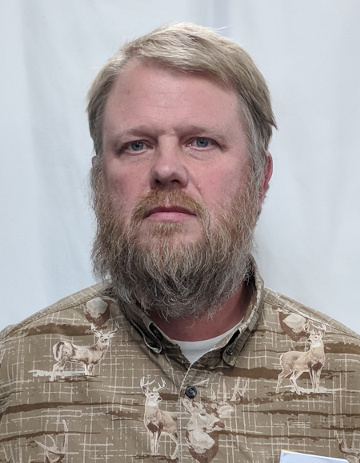Like   Tweet   +1
 /* styles */

## January 2018

 table div table+table+table+table div table{width:100%;padding:0}table div table+table+table+table div table img{width:96.23%;padding:0;float:none}table div table+table+table+table div table td{width:100%;padding:0 1.88% 18px}/* styles */## REMINDER...MACUL 2018 ConferenceThe MACUL Conference, Michigan's premier educational technology event, will be held March 7-9 at the DeVos Place in Grand Rapids, MI. Over 5,000 educators attend this conference annually and another year of record-level attendance is anticipated!

REMC1 recognizes the value of this conference and would like to assist our local schools in participating by providing the following accommodations:

Free Conference Registration & Bus Transportation to the conference for one person from your school district (Copper Country and Gogebic-Ontonagon ISD Schools only) Sign up here.

Discounted registration fee and bus fare for additional teachers to attend the conference from your district (We plan to reserve a charter bus again and subsidize the cost. We also coordinated with MACUL to receive a discount on registration through group pricing. Both of these items are dependent on reaching a minimum of 20 people from our region, though) Sign up here.

In order to receive the one complimentary registration and the discounted rates for additional teachers, REMC1 will need to register you with MACUL (DO NOT REGISTER ON MACUL's WEBSITE). Instead, please see our MACUL Registration & Bus Form. However, you will still need to book your hotel accommodation on your own and please visit MACUL's Conference Hotel Web page for reservations.

We are recommending the Holiday Inn and please reserve your hotel as soon as possible.
Additional information on the conference can be viewed at https://maculconference.org/ and questions can be directed to Steve Kass at steve@remc1.org

 table div table+table+table+table+table+table+table div table{width:100%;padding:0}table div table+table+table+table+table+table+table div table img{width:96.23%;padding:0;float:none}table div table+table+table+table+table+table+table div table td{width:100%;padding:0 1.88% 18px}/* styles */## Professional Development LineupWinter PD Events are here!
Registration is available on Wisdomwhere.
Wisdomwhere CCISD
Wisdomwhere GOISD
(Peer to CCISD to find and register)
_____________________

Make Learning Fun and Neat with Google’s G Suite
January 30, 2018--CCISD
February 2, 2018--GOISD
9 a.m. - 3 p.m.
Over the past two decades, Mid-continent Research for Education and Learning, known as McREL, researched strategies successful teachers employ in their classrooms. The strategies were categorized into nine different topics and placed into a framework for instructional planning. This framework starts with the essential task of creating the environment for learning which includes student engagement. Google’s G Suite offers several free resources that teachers can use to help engage their students, organize learning resources and address diverse learning needs. During this session, Google Certified Trainer, Steve Kass, will walk participants through some of these tools and how they can be best implemented utilizing the framework for instruction identified by McREL.

_______________________

February 9, 2018---GOISD
9 a.m. - 3 p.m.
Tech Academy is a program that allows our local schools to collaborate and discuss technology resources and implementation strategies. Each school in the Copper Country and Gogebic-Ontonagon ISD selects a teacher to represent them at the Tech Academy. During this workshop, the tech academy will be participating in the Michigan Educational Technology Specialists’ Winter Virtual Rally. The Virtual Rally will kick-off with a keynote speaker and will then break up into three roundtable discussion sessions to discuss new technology resources. After that, we will discuss ways we can implement these new resources in our schools!

_______________________

Virtual Reality in the Classroom
February 27, 2018--GOISD
March 1, 2018--CCISD
9 a.m. - 3 p.m.
The terms virtual reality and augmented reality are being discussed frequently these days but what do they actually mean and how do they relate to our K-12 classrooms? This workshop will explore virtual and augmented reality and some of the popular tools to implement these topics in your classroom. We will also debut the new REMC1 Virtual Reality Kit that can be checked out for your classroom for virtual and augmented reality experiences!

 table div table+table+table+table+table+table+table+table+table+table div table{width:100%;padding:0}table div table+table+table+table+table+table+table+table+table+table div table img{width:96.23%;padding:0;float:none}table div table+table+table+table+table+table+table+table+table+table div table td{width:100%;padding:0 1.88% 18px}/* styles */## Helpdesk TransitionREMC1 has been fortunate to have many great MTU students over the years that have worked to provide Tier1 of our Helpdesk. While we will continue to have part-time helpdesk interns, we are growing a second tier of full-time helpdesk staff. We now have 3 full-time helpdesk technicians available to help with your issues and troubles, in addition to 5 part-time interns, and our 5 full-time Tier3 Central Office Network & Support Engineers (as well as our 7 onsite System Support Engineers). Please continue to use our helpdesk for all your support needs, as we continue to grow and adapt to the ever-changing technology landscape.

 table div table+table+table+table+table+table+table+table+table+table+table+table+table div table{width:100%;padding:0}table div table+table+table+table+table+table+table+table+table+table+table+table+table div table img{width:96.23%;padding:0;float:none}table div table+table+table+table+table+table+table+table+table+table+table+table+table div table td{width:100%;padding:0 1.88% 18px}/* styles */## Meet Our Staff!We would like to introduce one of our newest staff members. Mark Somero was born and raised in the Copper Country, graduating from Dollar Bay High School in 1982. He graduated from NMU with a BS in Manufacturing in 1990. From 1990 to approximately 2003, Mark worked in several foundries in Wisconsin and Michigan specializing in alloy development. In 2005 he returned to school (Northeast Wisconsin Tech College) and obtained his AAS degree in Microcomputer Support with Network emphasis. After being away for approximately 26 years, he and his wife returned to the area. Mark enjoys the outdoors, hunting, fishing, camping, boating, hiking, etc. His other interests include gunsmithing, blacksmithing, and pyrotechnics. Welcome Mark!

 table div table+table+table+table+table+table+table+table+table+table+table+table+table+table+table+table div table{width:100%;padding:0}table div table+table+table+table+table+table+table+table+table+table+table+table+table+table+table+table div table img{width:96.23%;padding:0;float:none}table div table+table+table+table+table+table+table+table+table+table+table+table+table+table+table+table div table td{width:100%;padding:0 1.88% 18px}/* styles */## Teacher's CornerCheck out the updates to 21Things4Students...Maker: Sphero & Ozbots, Fake or Real News & Digital Storytelling has been totally redone!!

How important are password managers? Even their flaws double as reminders why you need one.

 table div table+table+table+table+table+table+table+table+table+table+table+table+table+table+table+table+table+table+table div table{width:100%;padding:0}table div table+table+table+table+table+table+table+table+table+table+table+table+table+table+table+table+table+table+table div table img{width:96.23%;padding:0;float:none}table div table+table+table+table+table+table+table+table+table+table+table+table+table+table+table+table+table+table+table div table td{width:100%;padding:0 1.88% 18px}/* styles */## ResourcesEduPaths is a free resource from TRIG created to centralize PD around the state. Find resources and get FREE SCECH hours!

Current REMC Connect (REMC Association of Michigan Newsletter)

Check out Discovery Streaming updates and enhancing instruction with streaming resources.

MeL is a great place for Michigan Teachers to find classroom resources. They have just recently launched MeL Teachers which offers eight centers that will help with site navigation and make important information easier to access.

 table div table+table+table+table+table+table+table+table+table+table+table+table+table+table+table+table+table+table+table+table+table+table div table{width:100%;padding:0}table div table+table+table+table+table+table+table+table+table+table+table+table+table+table+table+table+table+table+table+table+table+table div table img{width:96.23%;padding:0;float:none}table div table+table+table+table+table+table+table+table+table+table+table+table+table+table+table+table+table+table+table+table+table+table div table td{width:100%;padding:0 1.88% 18px}/* styles */## Connection Cornertable div table+table+table+table+table+table+table+table+table+table+table+table+table+table+table+table+table+table+table+table+table+table+table+table+table div table{width:100%;padding:0}table div table+table+table+table+table+table+table+table+table+table+table+table+table+table+table+table+table+table+table+table+table+table+table+table+table div table img{width:96.23%;padding:0;float:none}table div table+table+table+table+table+table+table+table+table+table+table+table+table+table+table+table+table+table+table+table+table+table+table+table+table div table td{width:100%;padding:0 1.88% 18px}/* styles */table div table+table+table+table+table+table+table+table+table+table+table+table+table+table+table+table+table+table+table+table+table+table+table+table+table+table+table div table{width:100%;padding:0}table div table+table+table+table+table+table+table+table+table+table+table+table+table+table+table+table+table+table+table+table+table+table+table+table+table+table+table div table img{width:96.23%;padding:0;float:none}table div table+table+table+table+table+table+table+table+table+table+table+table+table+table+table+table+table+table+table+table+table+table+table+table+table+table+table div table td{width:100%;padding:0 1.88% 18px}/* styles */table div table+table+table+table+table+table+table+table+table+table+table+table+table+table+table+table+table+table+table+table+table+table+table+table+table+table+table+table div table{width:100%;padding:0}table div table+table+table+table+table+table+table+table+table+table+table+table+table+table+table+table+table+table+table+table+table+table+table+table+table+table+table+table div table img{width:96.23%;padding:0;float:none}table div table+table+table+table+table+table+table+table+table+table+table+table+table+table+table+table+table+table+table+table+table+table+table+table+table+table+table+table div table td{width:100%;padding:0 1.88% 18px}/* styles */table div table+table+table+table+table+table+table+table+table+table+table+table+table+table+table+table+table+table+table+table+table+table+table+table+table+table+table+table+table+table div table{width:100%;padding:0}table div table+table+table+table+table+table+table+table+table+table+table+table+table+table+table+table+table+table+table+table+table+table+table+table+table+table+table+table+table+table div table img{width:96.23%;padding:0;float:none}table div table+table+table+table+table+table+table+table+table+table+table+table+table+table+table+table+table+table+table+table+table+table+table+table+table+table+table+table+table+table div table td{width:100%;padding:0 1.88% 18px}/* styles */table div table+table+table+table+table+table+table+table+table+table+table+table+table+table+table+table+table+table+table+table+table+table+table+table+table+table+table+table+table+table+table+table div table{width:100%;padding:0}table div table+table+table+table+table+table+table+table+table+table+table+table+table+table+table+table+table+table+table+table+table+table+table+table+table+table+table+table+table+table+table+table div table img{width:96.23%;padding:0;float:none}table div table+table+table+table+table+table+table+table+table+table+table+table+table+table+table+table+table+table+table+table+table+table+table+table+table+table+table+table+table+table+table+table div table td{width:100%;padding:0 1.88% 18px}/* styles */